# ⍵ = √ (2 * uK * g / L)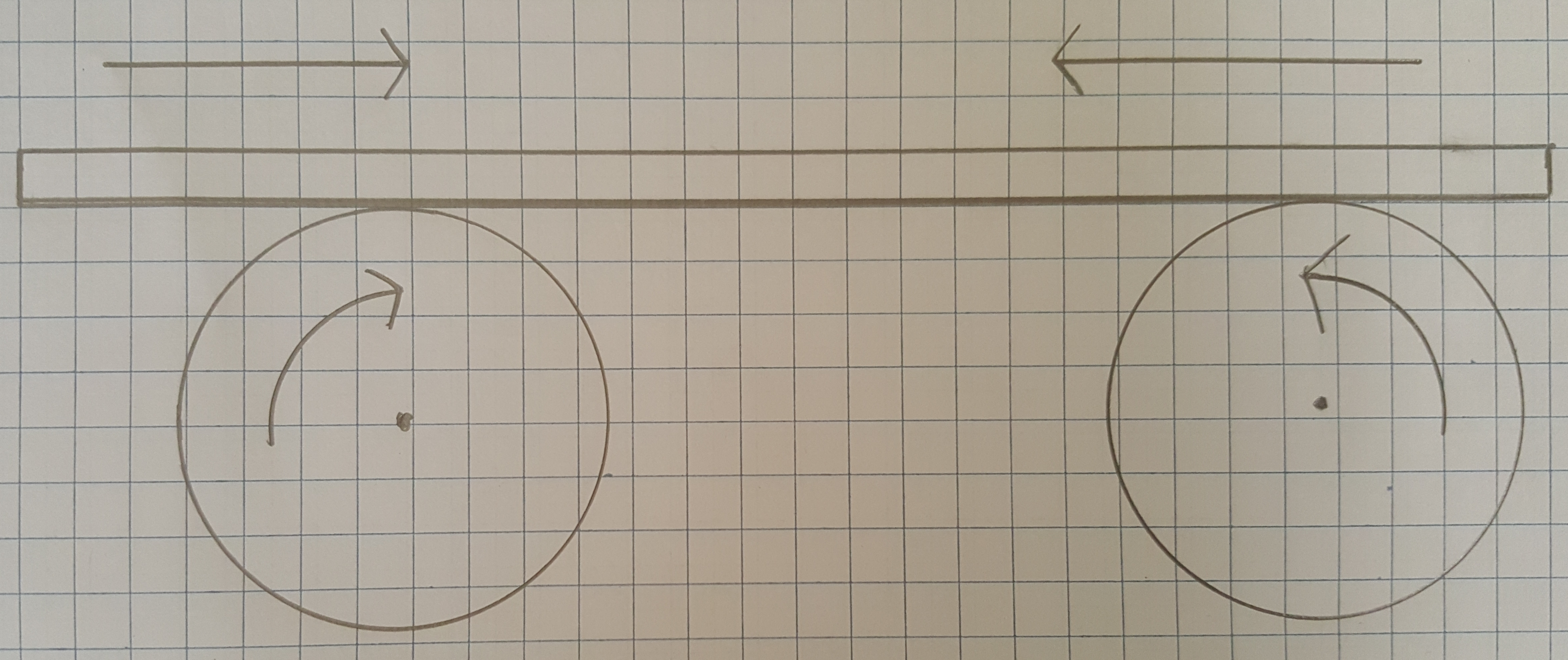# Introduction and overview

The oscillation frequency of a bar resting on two rollers turning towards each other is the square root of the coefficient of kinetic friction times twice acceleration of gravity (9.8 m/s^2) divided by the horizontal distance between the two rollers:

⍵  = √ (2 * uK * g / L)

This paper shows how the formula is computed, by summing forces and torques. There is data from tests and measurements of oscillation frequency using five different bars at varying wheel base lengths, that prove the formula is correct.

There are qualitative and quantitative proofs of this formula, recorded demonstrations, and data from empirical tests. There is background information about sources of information and where to find more information. There are explanations and videos about how to construct the test fixture, pictured below, used to model the problem.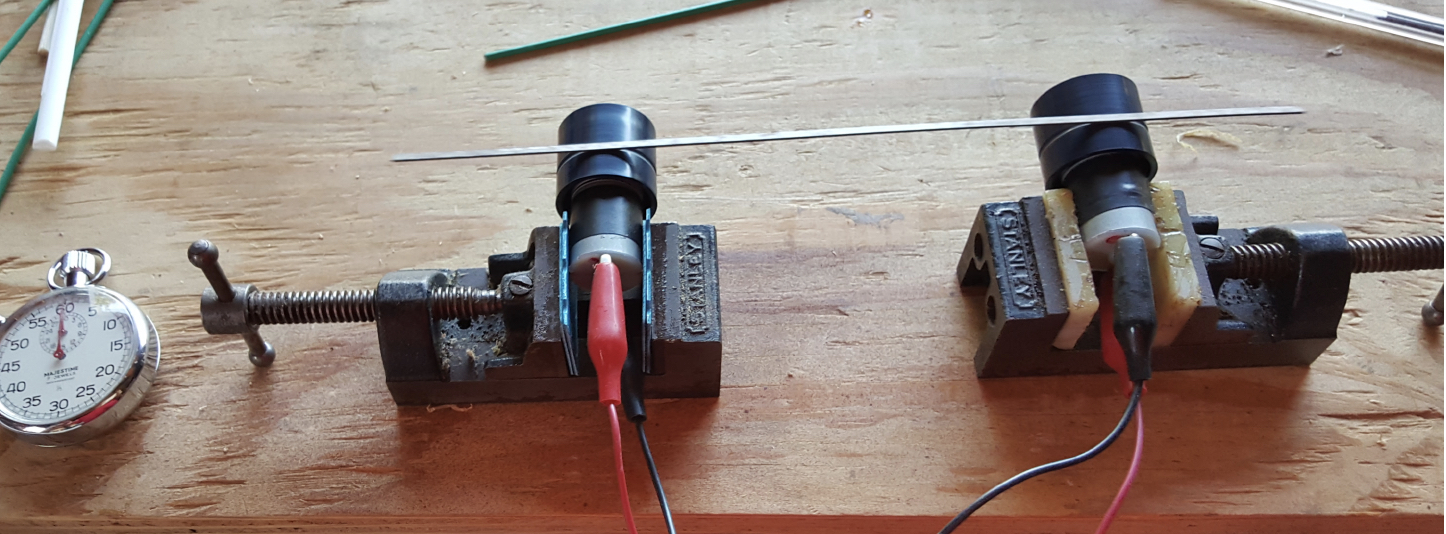The conclusion is that this formula is correct, accurate, and provable with a strong statistical correlation between wheel base and oscillation rate.

# How to compute oscillation frequency of the bar

There are five steps to computing oscillation frequency of the bar:

1. Sum vertical forces
2. Sum horizontal forces
3. Sum torques
4. Compute acceleration
5. Write equation of motion

It would be important to note that oscillation frequency of the bar is computed, and that forces and torques on the bar are summed.

# Sum vertical forces on the bar

There are two vertical forces in effect:

Normal forces, from the two rotors supporting the par, pointing up, in the positive Y direction, and
Gravity pulling the bar down, in the negative Y direction.

The bar is not moving up or down so the vertical forces sum to zero. The expressions are:

Nl + Nr - mg = 0
Nl + Nr =mg

The two normal forces are constantly changing. They are not equal. The bar is continuously moving back and forth and at almost any time more of the bar sits over one roller. This would mean the normal force of that rotor supporting more of the bar would be greater than the other rotor.

While the two normal forces are rarely equal and continuously changing, they sum to mass times gravity at all times.

# Sum horizontal forces on the bar

The only horizontal forces in effect are friction.

Friction pointing to the left. Negative horizontal direction.

When the bar is moving to the right, or in the positive X direction, it is driven by the rotor on the left. There is friction from the rotor on the right, opposing that motion. Friction opposes motion. The rotor on the right is opposing motion to the right, creating friction that points to the left, or in the negative X direction. The direction of friction is to the left, and it originates from the rotor on the right. The force is in the negative X direction.

Friction is equal to the coefficient of kinetic friction times the normal force. The expression for this is -uK*Nr

This friction is kinetic friction because the rotor on the right is sliding against the bar when the bar moves to the right.

Friction pointing to the right. Positive horizontal direction

When the bar is moving to the left, or in the negative X direction, it is driven by the rotor on the right. There is friction from the rotor on the left, opposing motion to the left. Friction opposes motion. The rotor on the left is opposing the motion to the left, creating friction that points to the right, or in the positive X direction. The direction of friction is to the right. It originates from the rotor on the left. The force of friction is in the positive X direction.

Friction is equal to the coefficient of kinetic friction times the normal force. The expression for this force is +uK*Nl

Sum of horizontal forces on the bar

The bar is moving nearly all the time. It is speeding up, slowing down, and changing direction. It is accelerating. The horizontal forces sum to mass times acceleration because the bar is moving horizontally:

uK * Nl - uK * Nr = ma
Nl - Nr = ma/uK

# Sum torques on the bar

There are four issues to consider when evaluating torques in a system:

1. Axis of rotation
2. Lever arm length
3. Magnitude of force on lever arm
4. Direction of force relative to lever arm

If there is a torque then there is an axis around which the object is moving or ‘wants’ to move because of the force on it. The best example of this is a person pushing a door on a hinge to close it. In this example the door hinge is the axis of rotation. The distance between door hinge and the person pushing the door is the length of the lever arm. The sine of the angle between the direction of force and plane of the lever is multiplied by magnitude of the force and length of the lever arm. The result of such an expression is the torque.

Once an axis has been decided on, all torques in the system must be evaluated as revolving, or attempting to revolve, around that single axis.

Six torques on the bar, in three pairs

1. Torques imposed by the normal forces on the bar
2. Torques imposed by the force of friction
3. Torques imposed by gravity

The torques imposed by normal forces on the bar are used, with the horizontal and vertical force summations, to isolate acceleration. The two other force pairs exist but are not used to compute oscillation frequency. All torques are presented because sources more reliable than this paper also tally up all torques.

Axis of rotation is the bar center of mass

A single axis of rotation must be identified in order to compute torques. There are several strategies for doing this. In this paper symmetry is used because it is generally a good approach in mechanical physics, electricity, and magnetism.

The center of mass of the bar is designated as the axis of rotation. The bar is assumed to have uniform linear mass density and therefore the center of mass is the linear center of the bar.

Locate the center of mass

The problem with choosing this axis of rotation is that the bar is constantly moving back and forth. In order to compute a torque the length of the arm and location of the axis must be correctly identified mathematically. This is accomplished by choosing the Y axis to intersect the middle of the wheel base, and using a variable “x” to represent how far the center of mass is from the Y axis.

If the center of mass is left of the Y axis, then x is a negative number. If the center of mass has oscillated to the right of the Y axis, then X is a positive number. The location of the axis can now be represented as being at location L/2 + x, where L is the length of the wheel base, L/2 is the middle of the wheel base, and x is distance between center of mass and center of wheel base. x is positive if the center of mass sits to the right of the Y axis, and x is a negative number of center of mass sits to the left.

Torques from normal force on the bar

There are two torques from normal forces on the bar. There is a clock-wise torque from the normal force of the left rotor, and a counter-clockwise torque normal force of the right rotor.

Clockwise torque from normal force of left rotor

If the axis of rotation is the bar center of mass, then the normal force from the left rotor is attempting to both push the bar up, and to rotate it in a clockwise direction. With torques, clockwise is a negative direction. This torque is represented as the normal force times the lever-arm length of L/2 - x. Choosing +x or -x is arbitrary but correct as long as the opposing torque uses L/2+x. This convention means, correctly, that L, the length of the wheel base, is L/2 - x + L/2 + x = 2 * L/2.

Torque = Force * Lever Arm Length * Sine of theta.

The length of the left lever arm is L/2 - x. The force is the left normal force Nl. The angle between the direction of force and lever arm is ninety degrees.  The sine of ninety degrees is one.

Torque from the left rotor is -Nl * (L/2 - x).

Counter-clockwise torque from normal force of right rotor

If the axis of rotation is the bar center of mass, then the normal force from the right rotor is attempting to both push the bar up, and rotate it in a counter-clockwise direction. With torques, counter-clockwise is a positive direction. This torque is represented as the normal force times the lever-arm length of L/2 + x. Choosing +x or -x is arbitrary but correct as long as the opposing torque uses L/2 - x.

The length of the right lever arm is L/2 - x. The force is the right normal force Nr. The angle between the direction of force and lever arm is ninety degrees.  The sine of ninety degrees is one.

Torque from the right rotor is +Nr * (L/2 + x).

These torques sum to zero. The bar is not rotating so the expression for torques from the normal force is:

Nr * (L/2 + x) - Nl * (L/2 - x) = 0

While this is the only torque used to compute oscillation frequency all professors and text books with explanations of this problem enumerate all the torques.

Torques from friction sum to zero

Forces of friction drive the bar horizontally in the direction opposing motion. The same force drives a torque attempting to rotate the bar around the axis, which is the center of mass. The direction of the frictional forces is towards the axis, meaning the angle between direction of force and lever arm is zero. The sine of zero is zero, so the torques in this case are zero because Force * Arm length * sine of zero degrees is zero.

Torque on the bar from gravity

Torques from gravity sum to zero. To understand torques from gravity in this system remember that the axis of rotation is the linear center of the bar.

The bar to the left side of the axis is pulled down by gravity, and if not obstructed by the roller would also pull the bar in a counter-clockwise direction. This is a positive torque. Similar to the formula used to sum torque from the normal forces, the torque from gravity, on the left side of the bar, is mg * (L/2 - x). The torque from gravity on the right side of the bar is mg * (L/2 + x).

The bar is not rotating so the torques from gravity sum to zero. The expression is:
mg(L/2 + x) - mg (L/2 - x) = 0

Explanatory note to those who disagree with this interpretation.

Some experts, including the professor who taught this problem, would disagree with the logic and principles used in this specific computation. An argument can be made that gravity acts through the center of mass of any object. The object in this case is a rod of uniform linear density. The center of mass is the linear center.

While I agree with this interpretation, I view it as a valid interpretation and not a technical fact. Gravity is operating equally on all parts of the rod. For this reason choosing a lever arm of any fixed length is flawed logic, but computing torques requires a fixed length arm.

The alternative interpretation, assuming gravity operates exclusively through the center of mass, which is the axis of rotation, would mean the arm length is zero. In this case the sum of torques from gravity is zero because length (zero) times angle times force is zero.

# Combine the equations. Isolate Nl, Nr, and acceleration

At this point there are written equations, summing vertical and horizontal forces, and torques around the bar center of gravity imposed by normal force. Respectively these equations are:

Vertical forces: Nl + Nr =mg
Horizontal forces: Nl - Nr = ma/uK
Torque from normal force: Nr * (L/2 + x) - Nl * (L/2 - x) = 0

The next steps in this process is to isolate acceleration. This is accomplished by rearranging the torques expression so that it has Nl + Nr, and Nl - Nr in it. That allows mg and ma/uK to be substituted in. When acceleration is in the equation it can be isolated and equated with w^2*x (w represents omega, angular frequency).

Steps to rearrange and substitute terms in the torques expression are as follows:

The original expression for torque from normal force of the rotors is:

Nr * (L/2 + x) - Nl * (L/2 - x) = 0

Multiply out all terms in the torques expression, giving:

Nr * L/2 + Nr * x - Nl * L/2 +  Nl * x = 0

Group terms with L/2 and x, giving:

L/2 * (Nr - Nl) + x * (Nl + Nr) = 0

The result now has Nl + Nr which can now be substituted for mg, resulting in:

L/2(Nr - Nl) + mg*x = 0

The expression has Nr - Nl, and what is needed is Nl - Nr, so some creative sign switching is in order. If the prefix term L/2 is given a negative sign, then signs on terms inside the brackets can be reversed. This preserves the value of the expression and permits using Nl - Nr.

L/2(Nr-Nl) = -L/2(Nl-Nr)

Because this is true the full expression becomes:

-L/2(Nl - Nr) + mg*x = 0

Now Nl - Nr can be substituted for ma/uK, giving:

-L/2 * ma / uK + mg*x = 0

Next, move mg*x to the other side and begin isolating acceleration, giving:

-L/2 * ma / uK = - mg*x

Mass exists on both sides and can be cancelled, giving:

-L/2 * a / uK = -g * x

Multiply both sides times 2 so the fraction goes away, giving:

-L * a / uK = -2 * g * x

Divide both sides by L, and multiply both sides by uK. Acceleration is now isolated
:

-a = 2 * g * x * uK / L

Acceleration is the second derivative of position with respect to time and can be represented as d^2x/dt^2

-a = - 2 * g * x * uK / L = d^2x/dt^2

Minus omega (angular velocity) squared times x is equal to d^2x/dt^2. This is an identity for SHM (single harmonic motion). This means that.

-a = - w^2x = - 2 * g * x * uK / L

Multiply the terms by negative one to make the negative sign go away, and:

w^2x = 2 * g  * x * uK / L

Take the square root of both sides and the result is omega, or angular frequency, or oscillation frequency is:

⍵  = √ (2 * uK * g / L)

This final expression is oscillation frequency of the bar. Computing this oscillation frequency is the objective of this problem.

Comparison with physical pendulum

The oscillation frequency of a physical pendulum is the square root of gravity over length:

⍵  = √ (g / L)

The oscillation frequency of the rod in this study contains the same term, the square root of g over L. The formulas differ by constants uK and 2. This would suggest the formula for an oscillating bar is either correct or at least in the proper direction.

Frequency and wheel base

The formula says that oscillation frequency varies inversely with the square root of wheel base (L). This is easily provable with the test fixture which allows positioning the rotors any distance apart. Test results in the following section strongly suggest the formula is correct.

Quantitative and qualitative proof of the formula was accomplished using a test fixture constructed for this specific purpose. It consists of two identical 3-Volt electric motors, each with a hard plastic rotor about one inch in diameter. The motors are wired to turn towards each other. The motors rotate at about 100 RPM which seems to be an ideal rate for this experiment.

Each motor is held in place using a small bench vise.

Motors are powered by an adjustable power supply, allowing tests of oscillation rate with varying RPM. Testing proved oscillation rate is independent of RPM.

The bench vices sit on a track allowing adjustment of wheel base from under three inches to more than meter. The track and vices ensure the rotors are aligned and level.

Five different bars were used for testing. They are:

Large bar. 23 13/16 inches long. Made of steel. Cut from a mattress frame. Rusty, scratchy surface. Weighs about three ounces.

Short bar. Eleven inches long. Same material as bar #1. These two bars were a single bar cut in two pieces. Specifically selected to test whether oscillation frequency is independent of mass as indicated by the formula under test. Tests prove in fact that oscillation rate is independent of mass.

Wood stick. Eleven inches long, one eighth inch in diameter. Very light. Smooth surfaces. Formerly a shish kabab skewer.

Thin bar. Nine and seven eighths inches long. Light, very thin, less than an eighth of an inch wide. Rusty scratchy surface. Found on the ground at a construction site. Weighs about a quarter of an ounce.

Plastic rod. Fifteen and thirteen sixteenth inches long, three eights of an inch wide. Moderately light. Cut from the long segment of a clothes hanger. Smooth surface.

Further details of fixture construction and links to video of the device in operation are in appendix 1 of this document.

# Qualitative proof

The video recording at this URL https://www.youtube.com/watch?v=XMoAaabO78U shows demonstrations varying the wheel base of with different bars. It is plainly visible that extending the wheel base causes oscillation frequency to decrease. Bars made of varying materials such as iron, plastic, and wood were used; with lengths between 9 and 24 inches.

Measurements of wheel base and oscillation rate are detailed in the following section on quantitative proofs.

Before measurements of wheel base and oscillation rate were taken, a video was made recording some qualitative proof of the expression under test. The video can be seen at: https://www.youtube.com/watch?v=XMoAaabO78U

The video of these tests proved an expected result and showed some anomalous and unexpected results.

As wheel base is extended, the oscillation rate clearly goes down. This was proven using five different bars of varying material, mass, dimension, and smoothness.

When the wheel base approaches length of the bar, in several cases the oscillation rate went back up.

Oscillations are most varied with a very short wheel and the heavier bars bounced around.

Oscillations are most consistent, smooth, and least varied when the wheel base is about half the length of the bar. At this position half the bar is between the rotors, and a quarter of the length of the bar extends beyond the right and left rotors.

# Quantitative proof

Test results prove that the relationship between oscillation rate and square root of wheel base is inverse linear with very strong R-Squared values from five different experiments.

Best fit analysis

The best fit analysis proves a linear inverse relationship between oscillation rate and square root of wheel base.

The following five plots prove the equation is correct and accurate. The graphs are each plot a magnitude one Matlab polyfit of the test data, overlaid with a plot of oscillation rate against the square root of wheel base. The R-Squared value for each pair of vectors is also shown.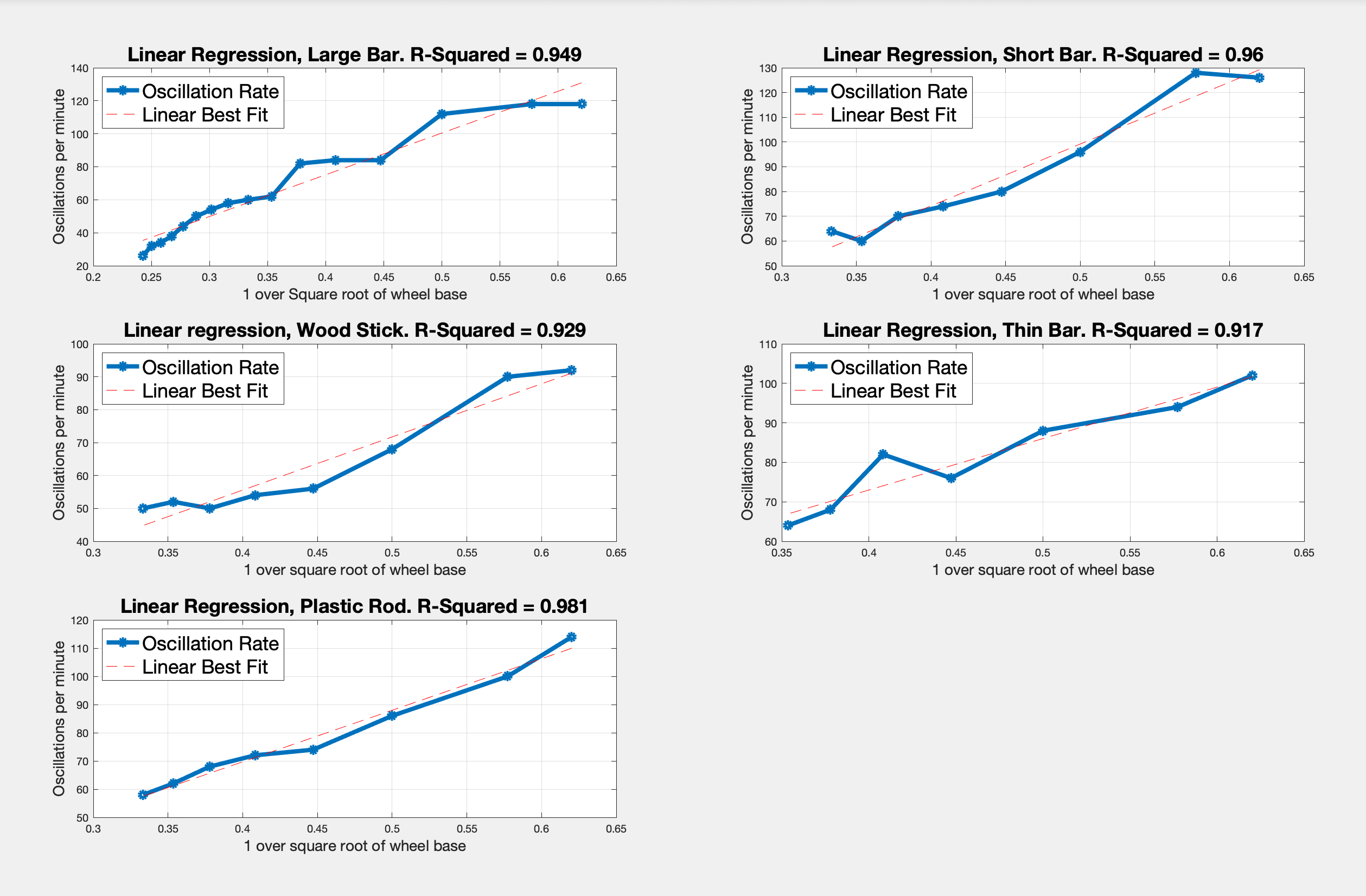With one exception the R-Squared values are all over 90%. This is high enough to cause suspicion among some statisticians who would consider such a strong correlation too good to be true. This information is a simple linear regression with a small number of data points for each test. There are five independent tests so veracity of the numbers is reasonable.

Readers of this white paper are free to download the test data. It is in CSV format and accessible at this URL:

http://www.embeddedlinuxtestengineer.com/TableOfResults.csv

The Matlab code used to generate these reports and graphs is also at the same web site, and can be downloaded from this URL:

http://embeddedlinuxtestengineer.com/hb_SHM.m

Following are discussions of the best fit analysis, plotting oscillations against wheel base, and an overview of the raw test data.

# Plots of oscillations and wheel base

The next set of four graphs is a rendition of all test results. The first row shows oscillations vs wheel base. The second row shows oscillations vs the square root of the wheel base. A visual inspection of these shows there is an inverse relationship between wheel base and oscillation rate.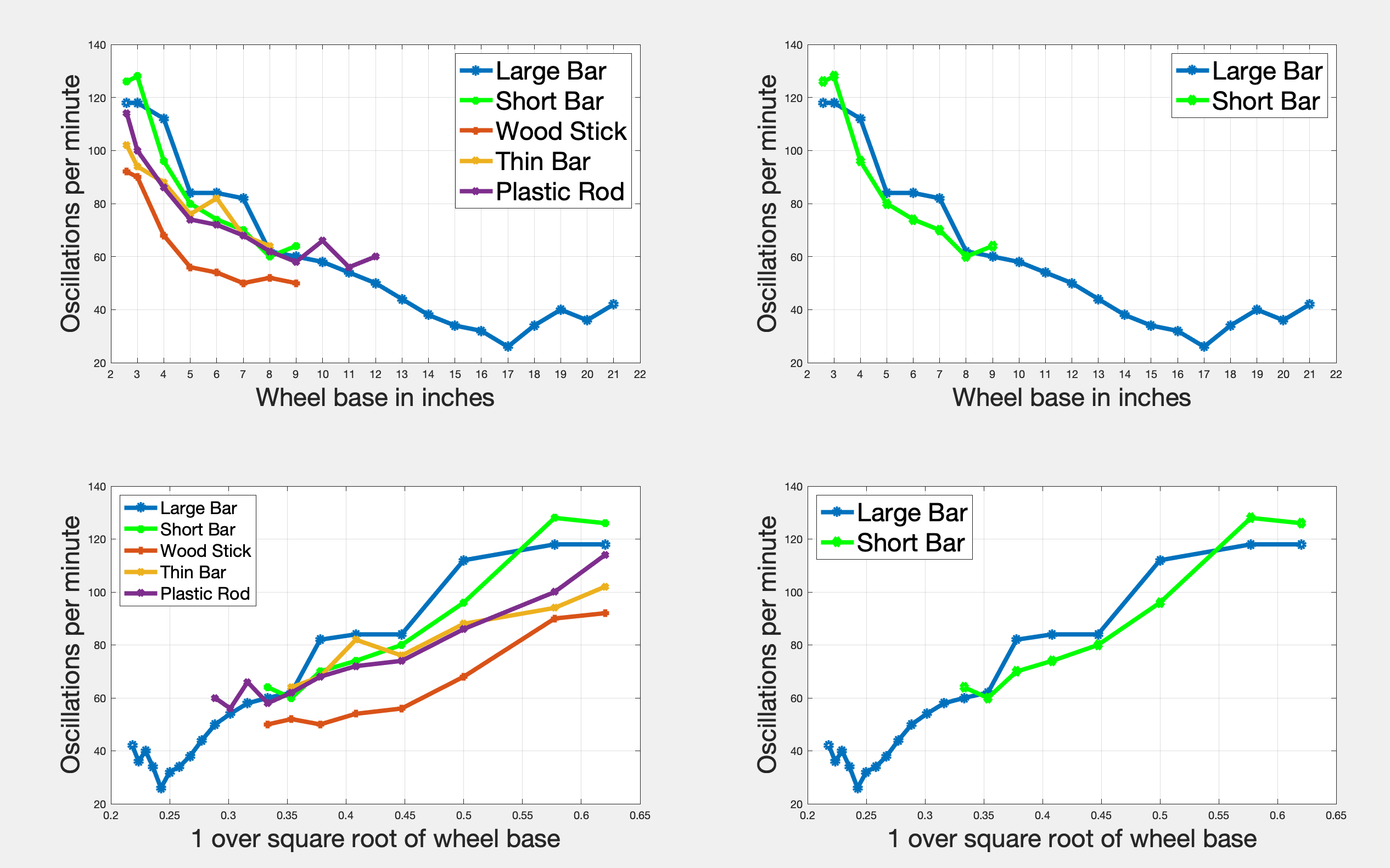The two graphs on the left are plots from tests recording oscillations of five different bars:

Large bar. 23 13/16 inches long. Made of steel. Cut from a mattress frame. Rusty, scratchy surface.

Short bar. Eleven inches long. Same material as bar #1. These two bars were a single bar cut in two pieces. Selected specifically to test whether the oscillation frequency is independent of mass as indicated by the formula under test.

Wood stick. Eleven inches long, one eighth of an inch in diameter. Very light. Smooth surfaces. Formerly a shish kabab skewer

Thin bar. Nine and seven eighths inches long. Light, very thin, less than an eighth of an inch wide. Rusty scratchy surface. Found on the ground at a construction site.

Plastic rod. Fifteen and thirteen sixteenth inches long, three eighths of an inch wide. Moderately light. Cut from the long edge of a clothes hanger. Smooth surface.

The two graphs on the right plot test data from measurements of two bars of identical material and different length. They were one bar, taken from a mattress frame, then cut in two pieces. Oscillation frequency according to the equation under test is independent of mass. The expected result is that oscillation rate of the two bars is the same and the graphs prove this is true.

Look at the test data

The following spreadsheet shows the raw data from test results. The first column shows the wheel base of the fixture in inches. The shortest possible wheel base is 2 9/16 inches. Oscillations were then counted after expanding the wheel base in one inch intervals.

 Wheel Base in Inches Large Bar 23 13/16” Short Bar 11” Wood Stick 11” Thin Bar 9 7/8” Plastic Rod 15 3/16” 2 9/16” 59 63 46 51 57 3 59 64 45 47 50 4 56 48 34 44 43 5 42 40 28 38 37 6 42 37 27 41 36 7 41 35 25 34 34 8 31 30 26 32 31 9 30 32 25 falls off 29 10 29 falls off falls off 33 11 27 28 12 25 30 13 22 falls off 14 19 15 17 16 16 17 13 18 17 19 20 20 18 21 21 22 falls off

Rotors turn at 100 revolutions per minute. Oscillation rate does not vary with RPM of the motors. Speed of the rotors causes the bar to move farther back and forth, equivalent to increasing amplitude, but this does not change the rate of oscillation.

The data first column shows oscillations in thirty seconds for the “large” bar, with a wheel base ranging from 2 9/16 inches to 22 inches. Beyond that the 23 13/16 inches the bar falls off the rollers. The numbers show an oscillation rate of 59 for the shortest wheel base, 21 for the longest wheel base, and minimum of 13 at seventeen inches.

Oscillation rate decreases as the wheel base is expanded up to seventeen inches. Beyond that point oscillation rate increases. This anomaly has been seen in tests with two different bars. The formula under test does not account for this. So far a plausible explanation has not been found.

In order to statistically account for this anomaly and to be consistent with good scientific method, these four anomalous statistics were simply dropped from the goodness of fit analysis. :) With these four figures included in the R-Squared analysis the R-Squared value would be 0.894 rather than 0.973.

The second data column shows oscillations of the ‘short’ metal bar, made of the same material as the ‘long’ bar, and is tested with the intention of proving oscillation frequency is independent of mass. This bar is eleven inches long. At the shortest wheel base it oscillates 63 times in half a minute, and as the wheel base is extended to ten inches the oscillation rate declines.

The third data column shows oscillations of an eleven inch wood stick. It is very light with a relatively smooth surface. The oscillation rate declines from a maximum of 47 at the shortest wheel base, and declines to a rate of about 25 when the wheel base is seven inches. From seven to ten inches in wheel base the oscillation rate does not change.

The fourth data column shows oscillations of a very thin metal bar that has a lot of rust on it. Oscillations decrease in frequency as the wheel base is extended.

The fifth data column shows oscillations of a thick light rod of plastic that is 15 3/16 inches long. These measurements exhibit a pattern similar to that of the large bar. With the shortest wheel base oscillations are rapid. As the wheel base is extended, oscillations slow down until a wheel base of ten inches is reached, and then the rate goes up. This result was seen in some tests. These final three numbers were removed from the best fit analysis, but R-Squared values with and without the anomalies are presented. If these three numbers were included in the R-Squared computation, the R-Squared value would be 0.838 instead of 0.926.

# Margin of error

The estimated margin of error in oscillation counts is three to five percent above and below the numbers shown, explained as follows:

All oscillation figures shown are the average of five measurements. Actual numbers varied by up to five percent above and below that mean.

It is inherently difficult to count oscillations by inspection and this may contribute to the margin of error.

Oscillation of all rods tested was inconsistent and uneven, and for this reason an average of five measurements is recorded in this paper.

Oscillation of the ‘long’ and ‘short’ bars at two shortest wheel base measurements was especially erratic.

The estimated margin of error in wheel base length is one sixteenth of an inch.

# Summary and conclusions

Oscillation frequency of a horizontal bar on two rollers turning towards each other is:

⍵  = √ (2 * uK * g / L)

This formula is computed using a summation of horizontal forces, vertical forces, and torques; followed by some creative algebra.

The formula is correct, accurate, and empirically provable.

Visual inspections of experiments indicate this formula is correct.

Empirical tests prove the formula is correct. This is best shown in the five graphs of best fit analysis. All R-Squared values are above 0.835 and most are above .926, indicating a very strong correlation.

Oscillation rate is independent of mass of the bar and speed of the rotors.

The formula also exhibits a direct square root relationship between the coefficient of kinetic friction and oscillation frequency. A suitable method of testing this has not been developed.

Analogues to oscillation of a simple pendulum

There are four analogues to oscillation of a simple pendulum in this experiment.

Oscillation rate of a simple pendulum is the square root of acceleration due to gravity divided by pendulum length. This differs from oscillation rate of the horizontal bar by a pair of constants.

Oscillation of both systems is independent of mass. This was proven for the horizontal bars in this experiment.

Limits of small angle approximation seem to apply:

Within the limits of small angle approximation, the initial angle of a simple pendulum has no effect on oscillation rate. This seems to be analogous to adjusting RPM of the rotors. A higher RPM produces greater amplitude and lateral velocity of the bar, but does not change oscillation rate.

If the angle of a simple pendulum exceeds that of a ‘small angle’ then the formula for oscillation rate no longer applies. This seems to be analogous to extending wheel base to nearly the length of the bar.

Testing the coefficient of kinetic friction

Tests varying the wheel base were successfully conducted, but experiments to prove the effect of varying the coefficient of kinetic friction were inconclusive. Two bars, with a very smooth surface and presumably a low value for coefficient of kinetic friction, being the plastic rod and wood stick, did not display significant difference in oscillation rate of the three metal bars with rough, rusted, and scratchy surfaces.

# Appendix 1 - Fixture construction

This section is written in response to various requests from students, teachers, and tutors interested in making one of these oscillating whirly-gigs. From a visual inspection of the most recent machine it is apparent this is not a painfully difficult project, and this section of the document is largely intended to point out caveats and pitfalls from prior experience so that interested people can make one without wasting time repeating some of my mistakes.

Please note there is a separate video covering this same topic of how to make the fixture. It can be seen at: https://youtu.be/o_54gWHb6BE

Fixture #5, seen in all demonstrations, and in the picture below, is made using a pair of three volt electric motors that were taken from a Zip Notes dispenser. The machines cost about \$15.00 each and can be ordered on line from zipnotes.com

The rotors came with the electric motor

These motors seemed to make for the perfect test fixture. At 100 RMP the rotation rate was just right. The rotors are made of plastic and produce the right amount of friction to make a visible consistent oscillation. Other test devices, with very smooth rotor surface or faster motor, were less successful.The motors are held in position using identical small Stanley bench vises, available at specialty hardware stores for about \$25.00 each. The vises sit on a track made of plywood allowing for precise alignment.

The motors are powered by an adjustable power supply with a range from 1.5 to 12 volts. While the motors are rated at 3 volts, nearly all tests are done with 1.5 volts. Increasing the voltage increases increases lateral speed, amplitude, and has no effect on oscillation rate. At very high RPM test bars do not seem to oscillate consistently. Supplying more than 3 volts to the motors for any length of time caused them to burn out.

The advantages of this system are:

• Near perfect RPM (100) for test purposes
• Excellent level of friction on the rotors
• Small groove on the rotors helps keep bars in place
• The wheel base is adjustable to any length, limited only by the fragile motors’ ability to support bars weighing more than about three ounces

The only disadvantages of this system are:

• The motors will wear out and require replacement after about 20 hours of use
• The motors are weak and require light bars. Three ounces is about the heaviest bar that will still allow a consistent sustained oscillation.

Fixture #4, seen in the video and following picture, was made with a pair of Erector Set electric motors.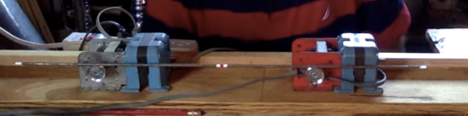While the geared motors supported a suitable RPM and produced consistent oscillations, the disadvantages are:

• Excessive motor vibration
• Motors overheat quickly
• Bars greater than a quarter inch in diameter do not fit
• Accurate alignment and wheel base adjustment, without a complex retaining fixture, is difficult

The remaining three fixtures, all made using electric motors from a cake mixer, had the same disadvantages, which were:

• Very smooth rotor surface did not produce a consistent observable oscillation
• Even at the slowest speed the RPM was much too high
• Excessive motor vibration

Fixture #3 is a pair of identical mixer motors mounted in separate frames allowing easy and simple adjustment of the wheel base. The motors are mounted in frames made from an erector set. The only advantage of this design is an adjustable wheel base, but the rotors turn much too fast and the hard metal surface of the rotors produced very little friction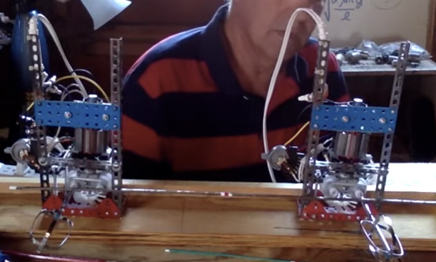Fixture #2 is a single mounted mixer motor, with all the disadvantages of fixture #3, plus a narrow and fixed wheel base.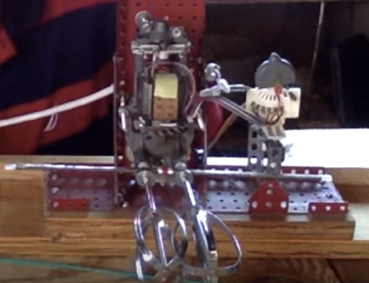Fixture #1 is a single mixer motor, mounted in a wood frame with an eighteen inch fixed wheel base. The rotors are turned using rubber bands as a belt. Like all the fixtures using a mixer motor, the RPM is too high and the rotor surfaces are too smooth.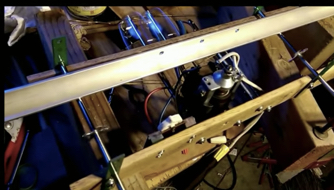## Cautionary notes re: fixture construction and sourcing parts

For those attempting to build one of these fixtures I would strongly advise caution when raiding your wife’s kitchen, vanity, or sewing room, for parts. The wood sticks were originally shish-kebab skewers and for some reason my wife was unhappy when I took them all and cut them up. She was especially unhappy when I began dissecting some of her cake mixers, and it did not help things when I bought two more for test fixtures, at prices significantly above the price of ones she originally had. Never mind that she still has at least two of them. Then for some reason she also became annoyed when I started taking all her white and red nail polish to mark the ends and center of gravity of all the bars used for testing. Nor did it help when I took a few large and apparently expensive knitting needles for testing as a bar. The dents and scratches just weren’t that bad. Then I took apart her favorite ZipNotes machine that had been on her desk. I just did not know she really used it. I thought she kept asking for paper refills for her friends.

For a while now I have been living in the garage and not because I want to. I just hope this all blows over before cold weather sets in.

Just giving you a heads up.

# Appendix #2 - Reference Information

This section is written for people who have asked where this problem came from and where to look for more information. It can be found in several text books, white papers, and recorded physics lectures from U.C. Berkeley.

The problem is illustrated on pages 377 and 378 in “Fundamentals of Physics” by Haliday, Resnick, and Walker, fifth edition. While there are many editions of this very well known physics text, the problem is found only in the fifth edition.

Copies of the pages from Haliday and Resnick are on Chegg, along with a vacuous regurgitation of the book’s solution. It is worth looking at this solution because the approach is different, using a different axis of rotation for computing torques, and using torques from gravity to help solve the problem.

I first heard of this problem from Dr. Achilles D. Speliotopoulos when he taught Physics 250 at Canada College in Redwood City, CA, during the fall 2015 semester. Dr. Speliotopoulos is a physics professor at U.C. Berkeley and occasionally moonlights at a local community college, which is how I had the privilege and pleasure of learning this. The problem caught my attention right away because it seemed to appear that a fixture demonstrating it could be easily built. At least I thought it would be easy…

Audio recordings of Dr. Speloptopoulos’ physics lectures at Berkeley can be heard at:

https://archive.org/details/ucberkeley_webcast_itunesu_915496122

The lecture dated 11/26/2014 contains his more eloquent description and solution. The preceding lecture, on 11/24/2014, is also worth listening to, with explanations of the equation of motion and other interesting problems with oscillation frequency.

The fixture Dr. Speliotopoulos uses to demonstrate the problem can be seen at:

http://berkeleyphysicsdemos.net/node/47

https://arxiv.org/pdf/0907.3378.pdf

While I found the paper less than illuminating it contains reference to another physics text that reportedly describes the problem.

An explanation of the problem, and an alternate solution, is presented by Physics Galaxy, at:

# Appendix #3 - Hyperlinks to test data, Matlab code, and videos

Soft-copy of the test data seen on page 19 can be downloaded from:

http://embeddedlinuxtestengineer.com/TableOfResults.csv

The Matlab script used to generate the graphs and R-Squared values seen in this paper can be downloaded from:

http://embeddedlinuxtestengineer.com/hb_SHM.m

A pdf of this white paper can be seen and downloaded from:

http://embeddedlinuxtestengineer.com/OscillatingHorizontalBarProblem.pdf

A video by this author showing how the expression for oscillation rate is computed can be seen at:

A video by this author citing sources and references can be seen at:

A video by this author presenting the qualitative and quantitative proof of the expression can be seen at:

A video by this author about how to construct a suitable test fixture to demonstrate this problem can be seen at:

Contact information

If you have comments or questions regarding this paper or any aspect of the proof, fixture construction, or anything else please feel free to send a note and I will write back.

Many thanks,
-Dave Ge
Redwood City, CA
June, 2019
daveg@embeddedlinuxtestengineer.com